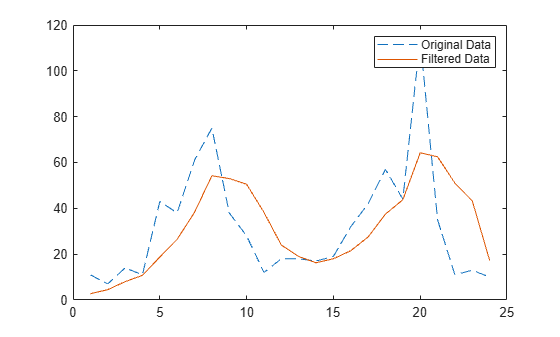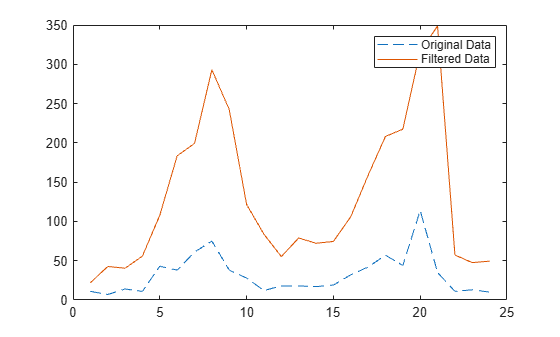Documentation

## Filter Data

### Filter Difference Equation

Filters are data processing techniques that can smooth out high-frequency fluctuations in data or remove periodic trends of a specific frequency from data. In MATLAB®, the `filter` function filters a vector of data x according to the following difference equation, which describes a tapped delay-line filter.

In this equation, a and b are vectors of coefficients of the filter, Na is the feedback filter order, and Nb is the feedforward filter order. n is the index of the current element of x. The output y(n) is a linear combination of the current and previous elements of x and y.

The `filter` function uses specified coefficient vectors a and b to filter the input data x. For more information on difference equations describing filters, see .

### Moving-Average Filter of Traffic Data

The `filter` function is one way to implement a moving-average filter, which is a common data smoothing technique.

The following difference equation describes a filter that averages time-dependent data with respect to the current hour and the three previous hours of data.

`$y\left(n\right)=\frac{1}{4}x\left(n\right)+\frac{1}{4}x\left(n-1\right)+\frac{1}{4}x\left(n-2\right)+\frac{1}{4}x\left(n-3\right)$`

Import data that describes traffic flow over time, and assign the first column of vehicle counts to the vector `x`.

```load count.dat x = count(:,1);```

Create the filter coefficient vectors.

```a = 1; b = [1/4 1/4 1/4 1/4];```

Compute the 4-hour moving average of the data, and plot both the original data and the filtered data.

```y = filter(b,a,x); t = 1:length(x); plot(t,x,'--',t,y,'-') legend('Original Data','Filtered Data')```### Modify Amplitude of Data

This example shows how to modify the amplitude of a vector of data by applying a transfer function.

In digital signal processing, filters are often represented by a transfer function. The Z-transform of the difference equation

`$\begin{array}{rcl}a\left(1\right)y\left(n\right)& =& b\left(1\right)x\left(n\right)+b\left(2\right)x\left(n-1\right)+...+b\left({N}_{b}\right)x\left(n-{N}_{b}+1\right)\\ & & -a\left(2\right)y\left(n-1\right)-...-a\left({N}_{a}\right)y\left(n-{N}_{a}+1\right)\end{array}$`

is the following transfer function.

`$Y\left(z\right)=H\left({z}^{-1}\right)X\left(z\right)=\frac{b\left(1\right)+b\left(2\right){z}^{-1}+...+b\left({N}_{b}\right){z}^{-{N}_{b}+1}}{a\left(1\right)+a\left(2\right){z}^{-1}+...+a\left({N}_{a}\right){z}^{-{N}_{a}+1}}X\left(z\right)$`

Use the transfer function

`$H\left({z}^{-1}\right)=\frac{b\left({z}^{-1}\right)}{a\left({z}^{-1}\right)}=\frac{2+3{z}^{-1}}{1+0.2{z}^{-1}}$`

to modify the amplitude of the data in `count.dat`.

Load the data and assign the first column to the vector `x`.

```load count.dat x = count(:,1);```

Create the filter coefficient vectors according to the transfer function $H\left({z}^{-1}\right)$.

```a = [1 0.2]; b = [2 3];```

Compute the filtered data, and plot both the original data and the filtered data. This filter primarily modifies the amplitude of the original data.

```y = filter(b,a,x); t = 1:length(x); plot(t,x,'--',t,y,'-') legend('Original Data','Filtered Data')```Oppenheim, Alan V., Ronald W. Schafer, and John R. Buck. Discrete-Time Signal Processing. Upper Saddle River, NJ: Prentice-Hall, 1999.